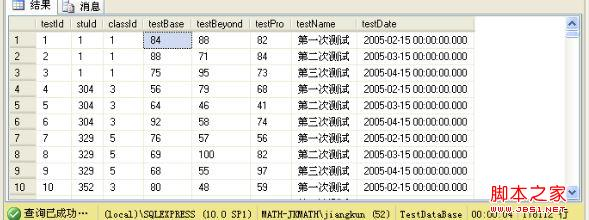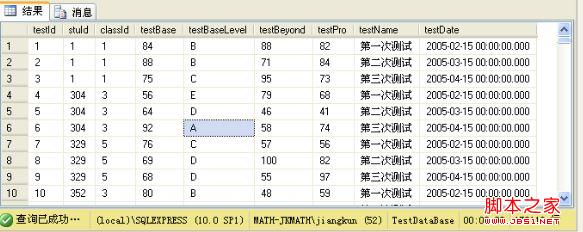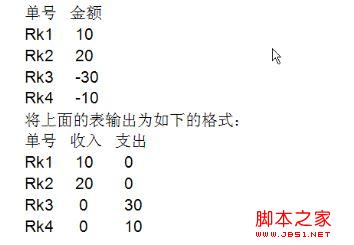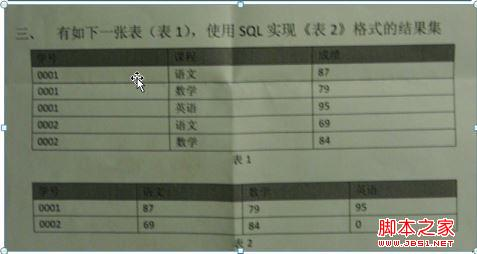1．Case函数的用法
(1)使用类似：switch-case与if-else if。
(2)语法：
case [字段]
when 表达式 then 显示数据
when 表达式 then 显示数据
else 显示数据
end
(3)百分制转换素质教育
1)如图：我们要将显示的数据转换成ABCDE，规则是90分以上显示A，80分以上显示B，以此类推。2)执行的SQL语句是：

Select ID,TestBase,
Case
When testBase>=90 then ‘A'
When testBase>=80 then ‘B'
When testBase>=70 then ‘C'
When testBase>=60 then ‘D'
Else ‘E' end as testBaseLevel,
testBeyond,testDate from Score

3)最后的执行结果如图所示：(4)注意：
1)写case对应的就写上end。
2)end后面跟别名(case与end之间相当于一个字段(对象))
(5)和C#的switch-语法作比较
1)C#
Switch(变量)
{
Case 常量1：结果1;break;
Case 常量2：结果2;break;
Default :默认结果;break;
}
2) SQL
SQL语法我在上面写了，可以对比看一下。
(6)对应的if-else if语法
1) C#
If(表达式1) {结果1;}
else if(表达式2) {结果2；}
else {默认结果;}
2)SQL Server
case
when 表达式1 then 显示1
when 表达式2 then 显示2
else 默认显示
end
3)举例说明，如果我们存放性别的时候在数据库中是用f,m标识的，现在我们想要用男女标识，SQL语句如下：

Select ID,Name,stuSex,
case
when stuSex='m' then ‘男'
when syuSex='f' then ‘女'
else ‘其它'
end as stuSexType,
stuDate from Student。

(7)练习案例：
1)在数据库中执行这段代码：

use Test
go
create table PracticeTest
(
number varchar(10),
amount int
)
insert into PracticeTest(number,amount) values('RK1',10)
insert into PracticeTest(number,amount) values('RK2',20)
insert into PracticeTest(number,amount) values('RK3',-30)
insert into PracticeTest(number,amount) values('RK4',-10)

2)实现的效果如下：3)可以看出，首先select中应该有三个字段，并且将数据大于0的放到收入中，那么另一个为0，并且将小于0的放到支出里面，另一个为0，下面我们写实现的SQL语句：

select number as 单号,
case
when amount>0 then amount
else 0
end as 收入,
case
when amount<0 then -amount
else 0
end as 支出
from PracticeTest

(8)一道面试题的练习：

1)如图：我们写出下面执行的代码，数据库大家自己建或者我在下面附加脚本了，大家制药执行一下即可：2)执行的SQL语句：

create table Score
(
学号 nvarchar(10),
课程 nvarchar(10),
成绩 int
)
insert into Score values('0001','语文',87)
insert into Score values('0002','数学',79)
insert into Score values('0003','英语',95)
insert into Score values('0004','语文',69)
insert into Score values('0005','数学',84)

3)实现功能的SQL语句的书写

select 学号,sum(
case
when 课程='语文' then 成绩
else 0
end) as 语文,sum(
case
when 课程='数学' then 成绩
else 0
end) as 数学,sum(
case
when 课程='英语' then 成绩
else 0
end) as 英语
from score group by 学号

••••